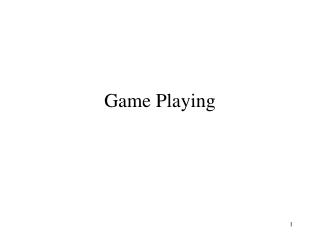# Game Playing - PowerPoint PPT PresentationDownload PresentationGame Playing

Game PlayingDownload Presentation## Game Playing

- - - - - - - - - - - - - - - - - - - - - - - - - - - E N D - - - - - - - - - - - - - - - - - - - - - - - - - - -
##### Presentation Transcript

1. Game Playing

2. Outline • Perfect Play • Resource Limits • Alpha-Beta pruning • Games of Chance

3. Games vs Search problems • Unpredictable opponent • use contingency plan • Time limits • only approximate solution • Approaches • algorithm for perfect play • finite horizon, approximation • pruning to reduce costs

4. Types of Games

5. Tic-Tac-Toe Example

6. Initial State starting configuration and whose move Operators legal moves Terminal Test Checks if game is over Utility Function Evaluates who has won and by how much Structure of a Game

7. Minimax

8. Minimax Algorithm function MINIMAX-DECISION(game) returns an operator for each op in OPERATORS[game] do VALUE[op] <- MINIMAX-VALUE(APPLY(op, game), game) end return the op with the highest VALUE[op] function MINIMAX-VALUE(state,game) returns a utility value if op TERMINAL-TEST[game](state) then return UTILITY[game](state) else if MAX is to move in state then return the highest MINIMAX-VALUE of SUCCESSORS(state) else return the lowest MINIMAX-VALUE of SUCCESSORS(state)

9. Properties of MiniMax • Complete ? • Yes (if tree finite) • Optimal ? • Yes, against optimal opponent • Time Complexity ? • O(bm) • Space Complexity ? • O(bm) (depth first exploration)

10. Resource Limits • Time complexity means given time limits • limited choice of solution • Approach • cutoff test (depth limit) • evaluation function (estimate of desirability of position)

11. Evaluation Functions • Normally weighted linear sum of features • Eval(s) = w1f1(s) + w2f2(s) + .. + wnfn(s)

12. Cutting off search • MinimaxCutoff identical to MinimaxValue except • TERMINAL? Replaced by CUTOFF? • UTILITY replaced by EVAL • In practice if bm = 106, b = 35 => m = 4 • 4-ply - human novice • 8-ply - typical PC or human master • 12-ply - Deep Blue, grand master

13. MAX 3 A1 A3 A2 <=5 MIN 3 X <=2 X<=2 <=14 A23 A13 A33 A11 A21 A31 A12 A22 A32 2 3 12 X 8 X 14 X 5 5 X X 2 Alpha-Beta pruning Example

14. Properties of Alpha-Beta • pruning doesn’t effect final result • Ordering improves efficiency of pruning • “perfect ordering”, time complexity O(bm/2) • doubles depth of search • In practice, time complexity O(b3m/4)

15. Alpha-Beta Algorithm function MAX-VALUE(state, game, alpha, beta) returns the minimax value of a state inputs: state, current state in game game, game description alpha, the best score for MAX along the path to state beta, the best score for MIN along the path to state if CUT-OFF(state) then return EVAL(state) for each s in SUCCESSORS(state) do alpha <- MAX(alpha, MIN-VALUE(s, game, alpha, beta)) if alpha >= beta then return beta end return alpha

16. Alpha-Beta cont. function MIN-VALUE(state, game, alpha, beta) returns the minimax value of a state if CUT-OFF(state) then return EVAL(state) for each s in SUCCESSORS(state) do beta <- MIN(beta, MAX-VALUE(s, game, alpha, beta)) if alpha >= beta then return alpha end return beta

17. Deterministic Games • Checkers:- • Chinook, 1994 • Chess • Deep Blue, 1997 • Othello • computers too good • Go • computers too bad

18. Non-deterministic Games • Chance adds difficulty • dice roll, deal of cards, flip of coin • ExpectiMax, like MiniMax • with additional chance nodes

19. Backgammon

20. expectimax(C) = sumi(p(di) maxs(utility(s))) C: chance node, di: dice roll p(di): probability of roll occurring maxs(utility(s)): max utility possible after dice roll Time Complexity O(bm nm) makes problems even harder to solve ExpectiMiniMax